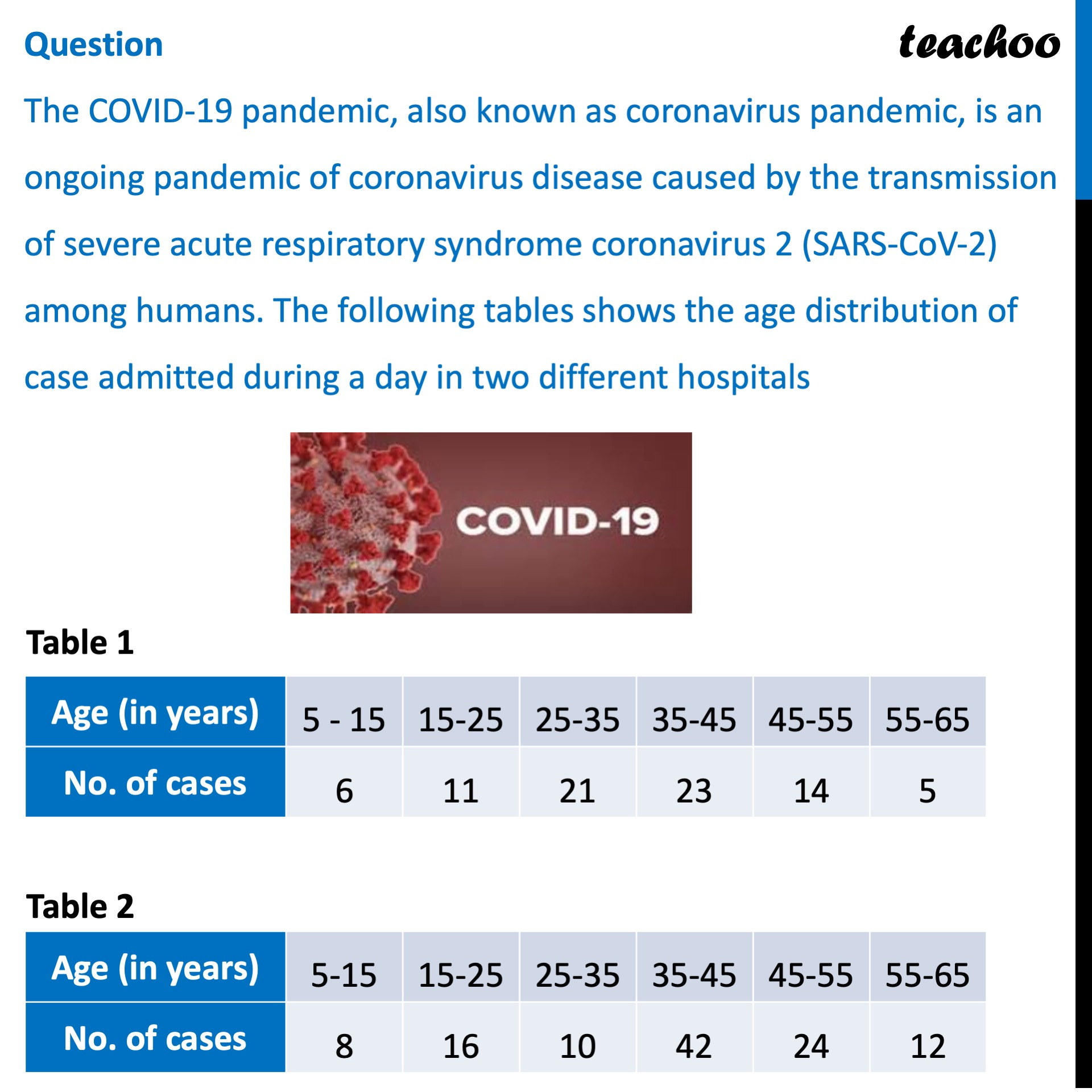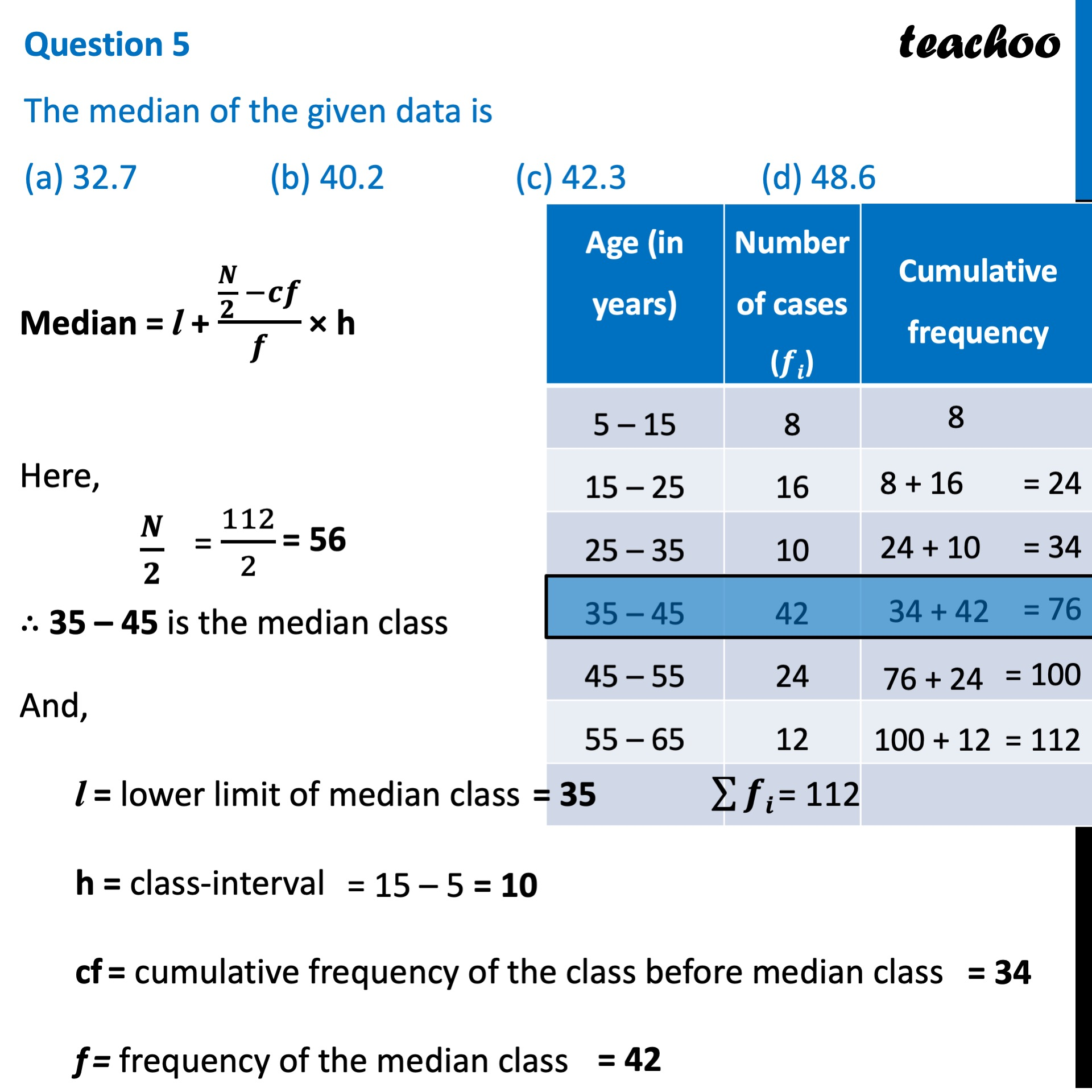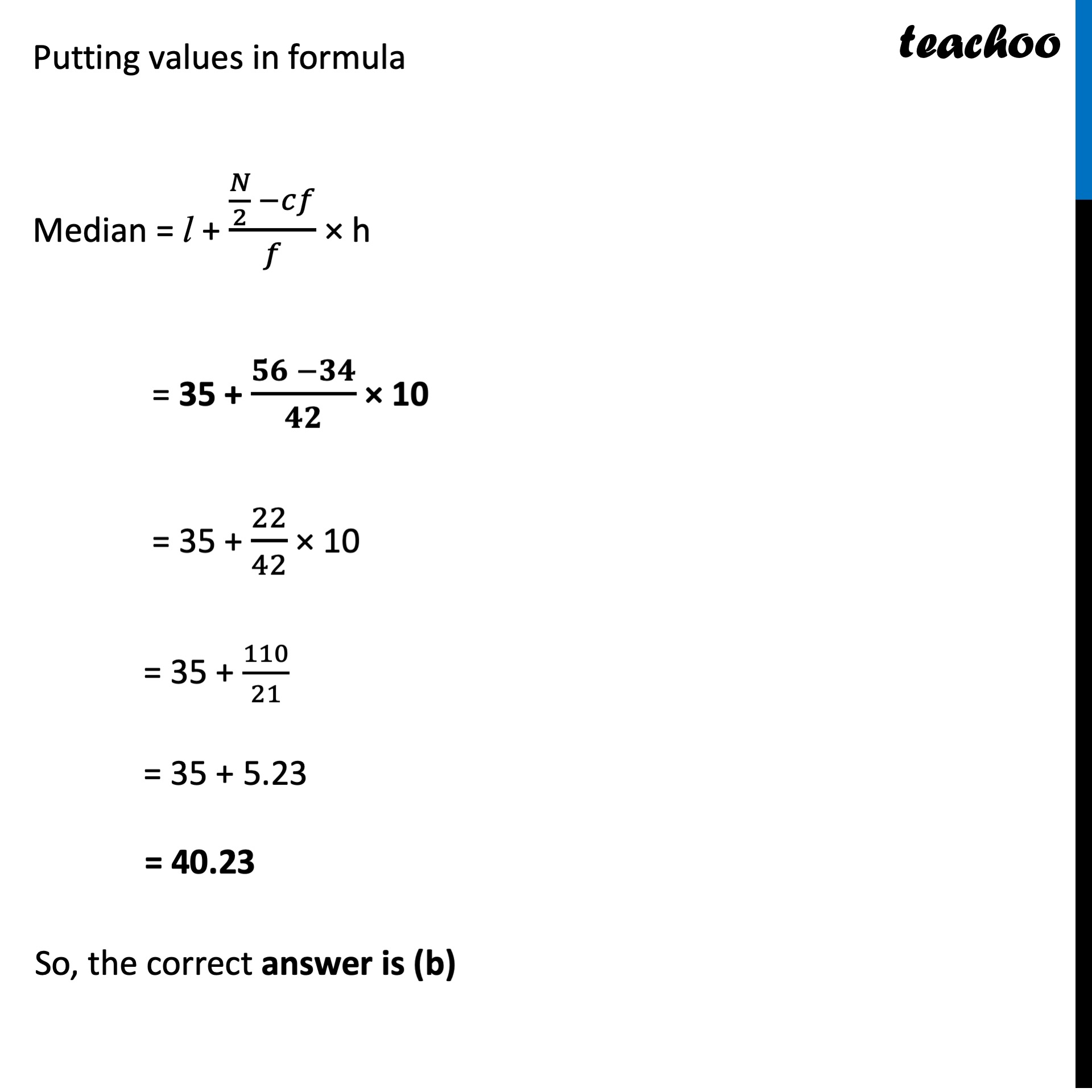Case Based Questions (MCQ)

Chapter 13 Class 10 Statistics
Serial order wise

## The COVID-19 pandemic, also known as coronavirus pandemic, is an ongoing pandemic of coronavirus disease caused by the transmission of severe acute respiratory syndrome coronavirus 2 (SARS-CoV-2) among humans. The following tables shows the age distribution of case admitted during a day in two different hospitals##Learn in your speed, with individual attention - Teachoo Maths 1-on-1 Class

### Transcript

Question The COVID-19 pandemic, also known as coronavirus pandemic, is an ongoing pandemic of coronavirus disease caused by the transmission of severe acute respiratory syndrome coronavirus 2 (SARS-CoV-2) among humans. The following tables shows the age distribution of case admitted during a day in two different hospitalsRefer to Table 1 Question 1 The average age for which maximum cases occurred is (a) 32.24 (b) 34.36 (c) 36.82 (d) 42.24(c) 36.82 Now, When we want to find average age, we find mean But, when we need to find average age of maximum cases, we need to find mode Finding Mode Mode = l + (𝒇𝟏 − 𝒇𝟎)/(𝟐𝒇𝟏 − 𝒇𝟎 − 𝒇𝟐) × h Modal class = Interval with highest frequency = 35 – 45 where l = lower limit of modal class h = class-interval f1 = frequency of the modal class f0 = frequency of class before modal class f2 = frequency of class after modal class Putting values in formula Mode = l + (𝑓1 −𝑓0)/(2𝑓1 −𝑓0 −𝑓2) × h = 35 + (𝟐𝟑 −𝟐𝟏)/(𝟐(𝟐𝟑) − 𝟐𝟏 − 𝟏𝟒) × 10 = 35 + 2/(46 − 35) × 10 = 35 + 2/11 × 10 = 35 + 1.818 = 36.818 ≈ 36.82 So, the correct answer is (c) Question 2 The upper limit of modal class is (a) 15 (b) 25 (c) 35 (d) 45(d) 45 Modal class is class with highest frequency Since 35 − 45 has highest frequency 23 ∴ It is the modal class And Upper limit of Modal Class = 45 So, the correct answer is (d) Question 3 The mean of the given data is (a) 26.2 (b) 32.4 (c) 33.5 (d) 35.4(d) 35.4 Mean(𝑥 ̅) = (∑▒𝒇𝒊𝒙𝒊)/(∑▒𝒇𝒊) = 2830/80 = 35.375 = 35.4 So, the correct answer is (d) Refer to table 2 Question 4 The mode of the given data is (a) 41.4 (b) 48.2 (c) 55.3 (d) 64.6(a) 41.4 Mode = l + (𝒇𝟏 − 𝒇𝟎)/(𝟐𝒇𝟏 − 𝒇𝟎 − 𝒇𝟐) × h Modal class = Interval with highest frequency = 35 – 45 where l = lower limit of modal class h = class-interval f1 = frequency of the modal class f0 = frequency of class before modal class f2 = frequency of class after modal class Putting values in formula Mode = l + (𝑓1 −𝑓0)/(2𝑓1 −𝑓0 −𝑓2) × h = 35 + (𝟒𝟐 − 𝟏𝟎)/(𝟐(𝟒𝟐) −𝟏𝟎 −𝟐𝟒) × 10 = 35 + 32/(84 −34) × 10 = 35 + 32/50 × 10 = 35 + 32/5 = 35 + 6.4 = 41.4 Question 5 The median of the given data is (a) 32.7 (b) 40.2 (c) 42.3 (d) 48.6(b) 40.2 Median = l + (𝑵/𝟐 −𝒄𝒇)/𝒇 × h Here, 𝑵/𝟐 ∴ 35 – 45 is the median class And, l = lower limit of median class h = class-interval cf = cumulative frequency of the class before median class f = frequency of the median class Putting values in formula Median = l + (𝑁/2 −𝑐𝑓)/𝑓 × h = 35 + (𝟓𝟔 −𝟑𝟒)/𝟒𝟐 × 10 = 35 + 22/42 × 10 = 35 + 110/21 = 35 + 5.23 = 40.23 So, the correct answer is (b)